# Electric Charge Chapter 21 Week1 Chapter 21 Electric

• Slides: 37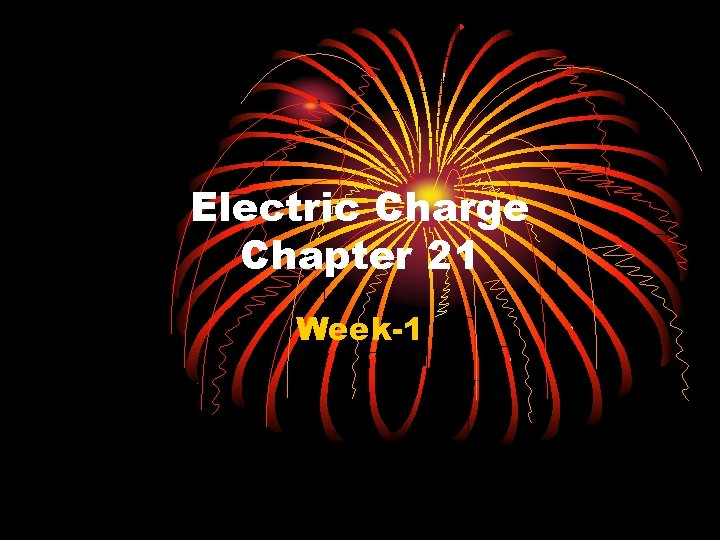Electric Charge Chapter 21 Week-1Chapter 21 Electric Charge In this chapter we will introduce a new property of matter known as “electric charge” (symbol q ). Moreover, we will describe the following properties of charge: - Types of electric charge - Forces among two charges (Coulomb’s law) - Charge quantization - Charge conservation (21 -1)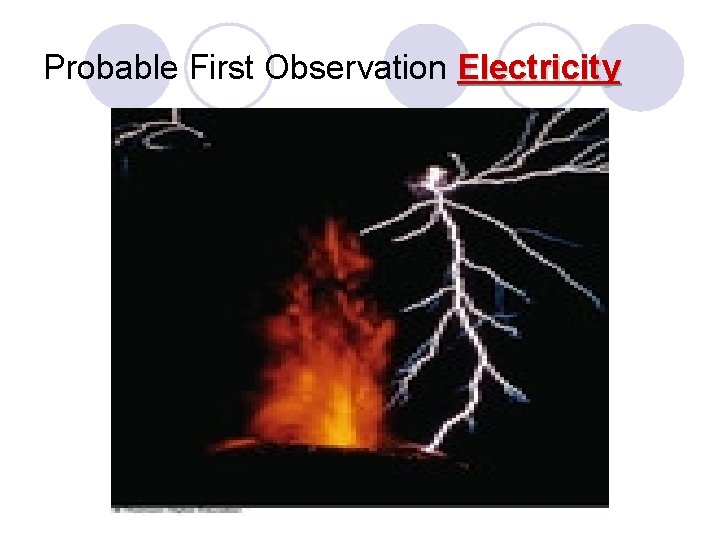Probable First Observation Electricity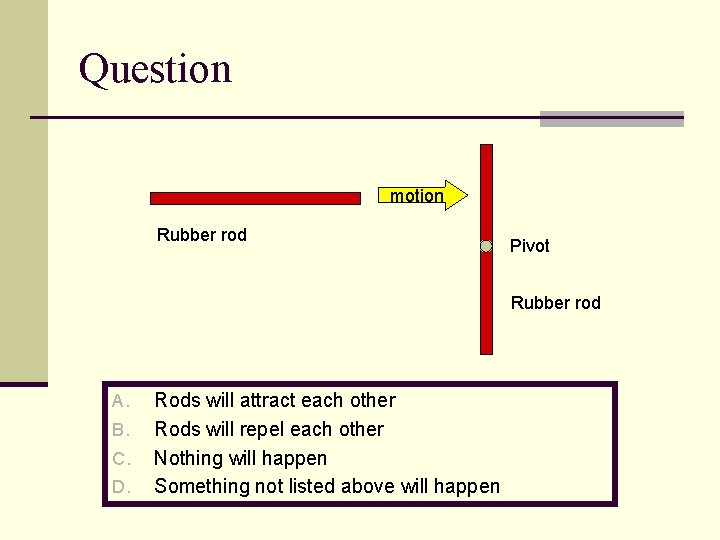Question motion Rubber rod Pivot Rubber rod A. B. C. D. Rods will attract each other Rods will repel each other Nothing will happen Something not listed above will happen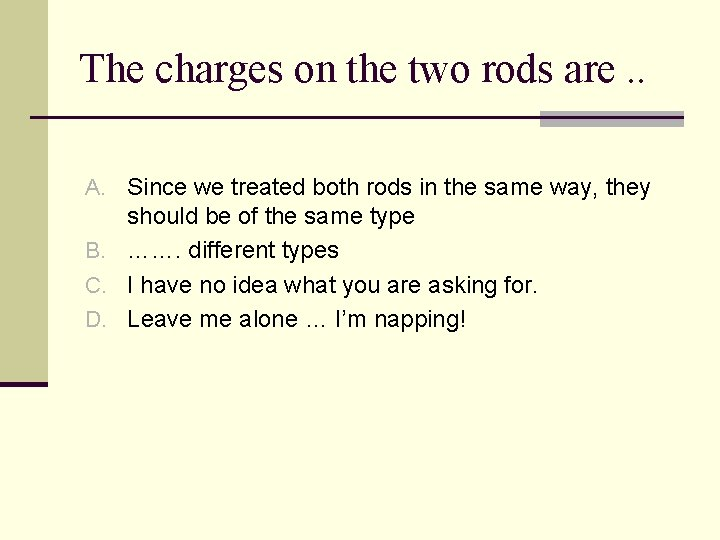The charges on the two rods are. . A. Since we treated both rods in the same way, they should be of the same type B. ……. different types C. I have no idea what you are asking for. D. Leave me alone … I’m napping!If you rubbed the rods longer and/or harder, do you think the effect that you see would be A. Stronger B. Weaker C. The sameIf the two rods are brought closer together, the force acting between them will get … A. Stronger B. Weaker C. The same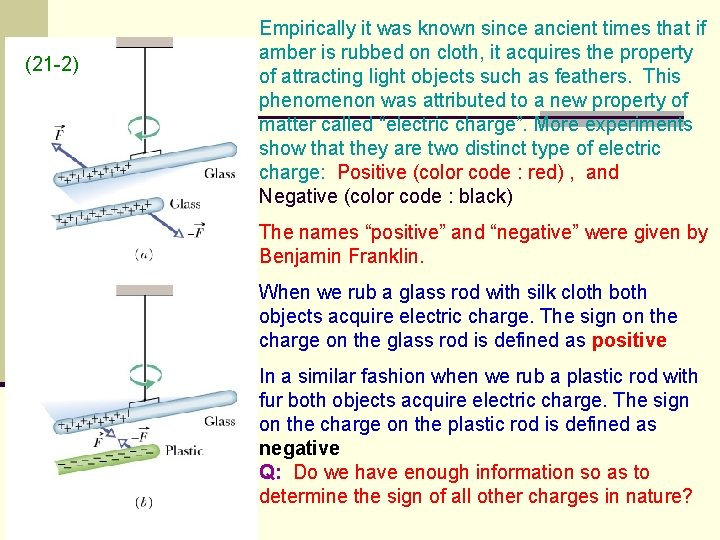(21 -2) Empirically it was known since ancient times that if amber is rubbed on cloth, it acquires the property of attracting light objects such as feathers. This phenomenon was attributed to a new property of matter called “electric charge”. More experiments show that they are two distinct type of electric charge: Positive (color code : red) , and Negative (color code : black) The names “positive” and “negative” were given by Benjamin Franklin. When we rub a glass rod with silk cloth both objects acquire electric charge. The sign on the charge on the glass rod is defined as positive In a similar fashion when we rub a plastic rod with fur both objects acquire electric charge. The sign on the charge on the plastic rod is defined as negative Q: Do we have enough information so as to determine the sign of all other charges in nature?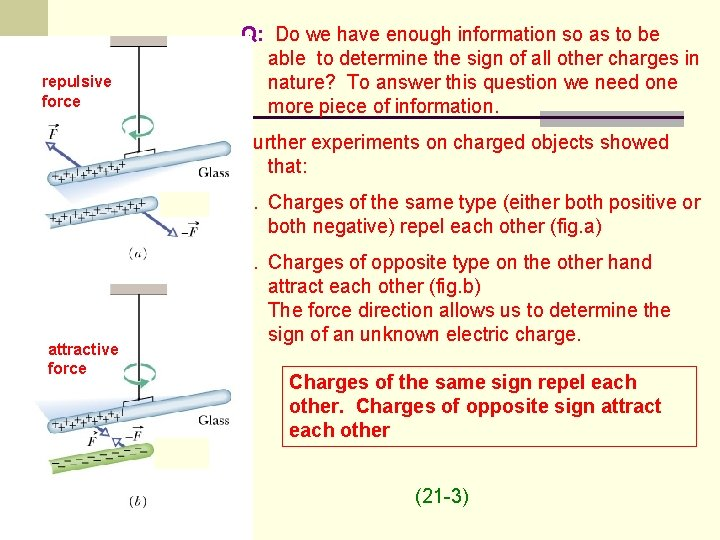repulsive force Q: Do we have enough information so as to be able to determine the sign of all other charges in nature? To answer this question we need one more piece of information. Further experiments on charged objects showed that: 1. Charges of the same type (either both positive or both negative) repel each other (fig. a) attractive force 2. Charges of opposite type on the other hand attract each other (fig. b) The force direction allows us to determine the sign of an unknown electric charge. Charges of the same sign repel each other. Charges of opposite sign attract each other (21 -3)What’s Going On? n All of these effects involve rubbing two surfaces together. n Or pulling two surfaces apart. n Something has “happened “to each of these objects. n These objects have a new PROPERTY n Other properties are mass, color n We call this NEW PROPERTY. ………CHARGE. n There seems to be two types of charge.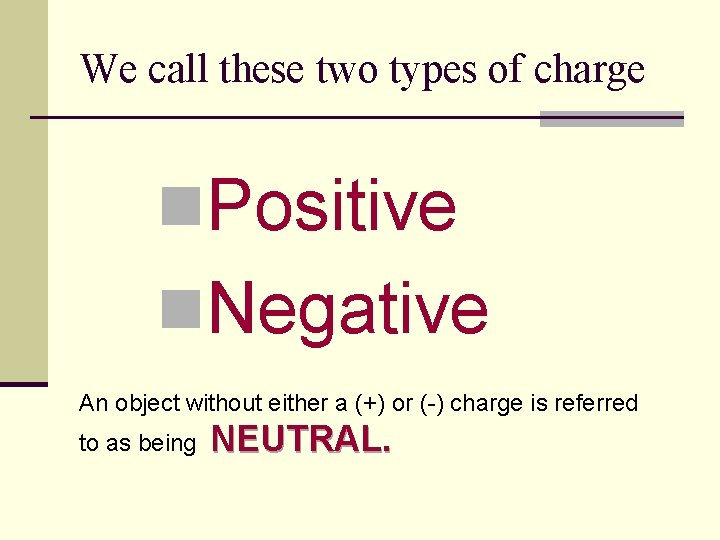We call these two types of charge n. Positive n. Negative An object without either a (+) or (-) charge is referred to as being NEUTRAL.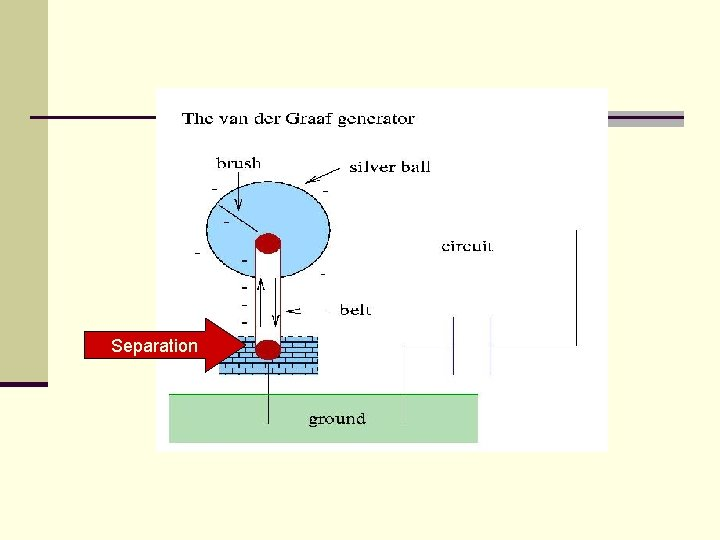SeparationAn Example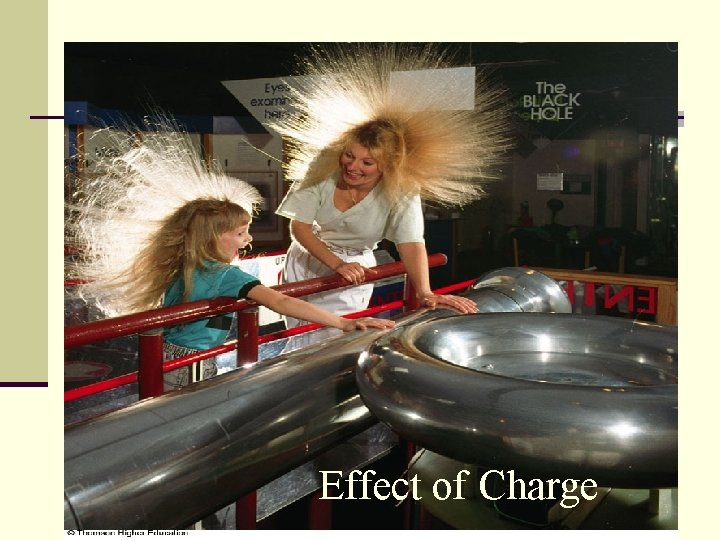Effect of Charge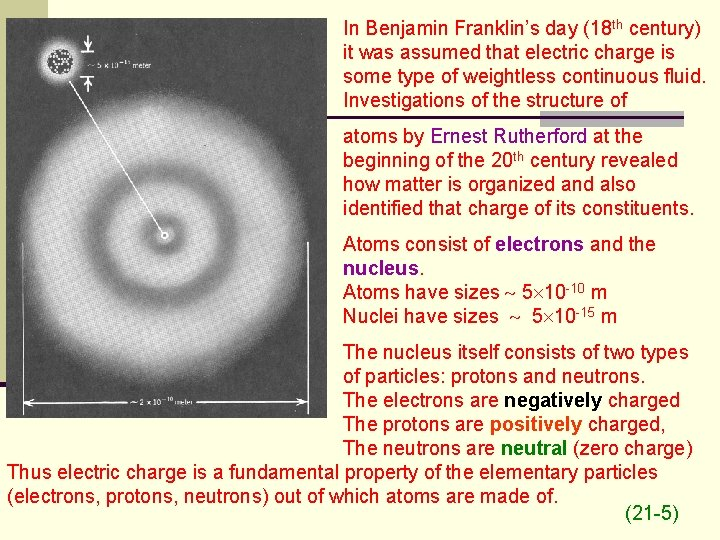In Benjamin Franklin’s day (18 th century) it was assumed that electric charge is some type of weightless continuous fluid. Investigations of the structure of atoms by Ernest Rutherford at the beginning of the 20 th century revealed how matter is organized and also identified that charge of its constituents. Atoms consist of electrons and the nucleus. Atoms have sizes 5 10 -10 m Nuclei have sizes 5 10 -15 m The nucleus itself consists of two types of particles: protons and neutrons. The electrons are negatively charged The protons are positively charged, The neutrons are neutral (zero charge) Thus electric charge is a fundamental property of the elementary particles (electrons, protons, neutrons) out of which atoms are made of. (21 -5)From whence this charge? ? ? Easily Removed +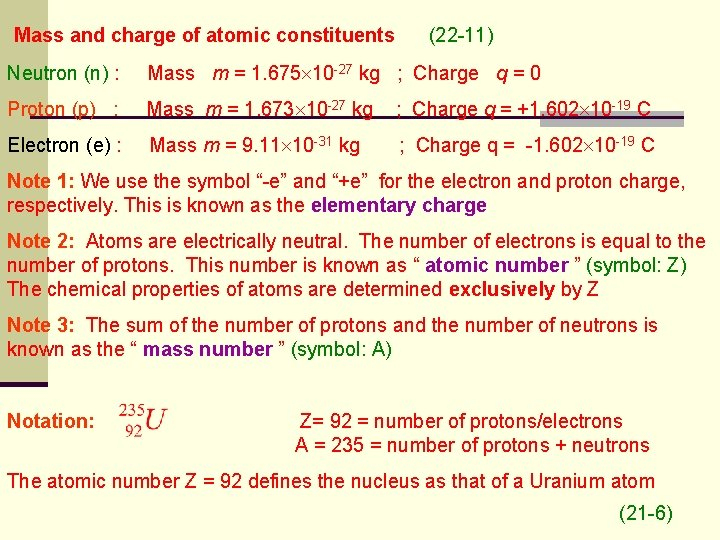Mass and charge of atomic constituents (22 -11) Neutron (n) : Mass m = 1. 675 10 -27 kg ; Charge q = 0 Proton (p) : Mass m = 1. 673 10 -27 kg ; Charge q = +1. 602 10 -19 C Electron (e) : Mass m = 9. 11 10 -31 kg ; Charge q = -1. 602 10 -19 C Note 1: We use the symbol “-e” and “+e” for the electron and proton charge, respectively. This is known as the elementary charge Note 2: Atoms are electrically neutral. The number of electrons is equal to the number of protons. This number is known as “ atomic number ” (symbol: Z) The chemical properties of atoms are determined exclusively by Z Note 3: The sum of the number of protons and the number of neutrons is known as the “ mass number ” (symbol: A) Notation: Z= 92 = number of protons/electrons A = 235 = number of protons + neutrons The atomic number Z = 92 defines the nucleus as that of a Uranium atom (21 -6)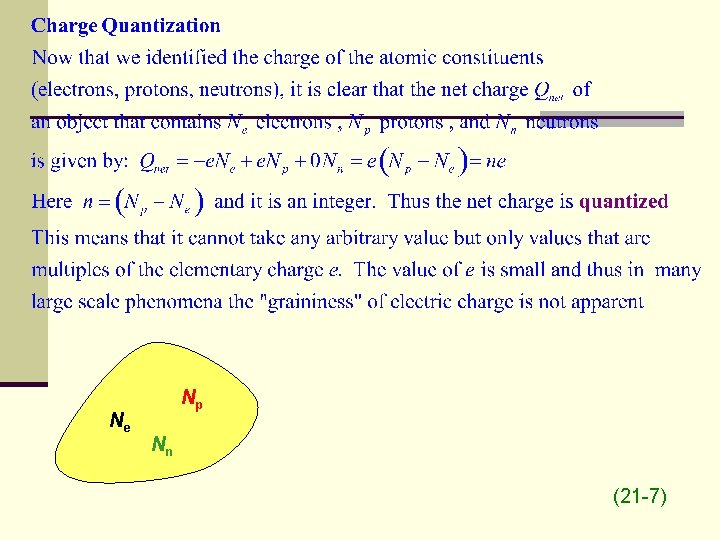Ne Np Nn (21 -7)silk Conservation of Charge glass rod silk - - - + + glass rod (21 -8)Materials + + n Two kinds of materials: n Insulators n n Conductors n n n Electrons and Protons are tightly bound to their positions. Hard to move them around. Electrons are easily removed and moved around. Electrons are said to be MOBILE charges. There are other kinds of materials that we will not discuss: semiconductors, semi-metals +Ways to charge an object n Transfer n Direct transfer n Polarization n InductionFig. a Fig. b Connection to ground (21 -11)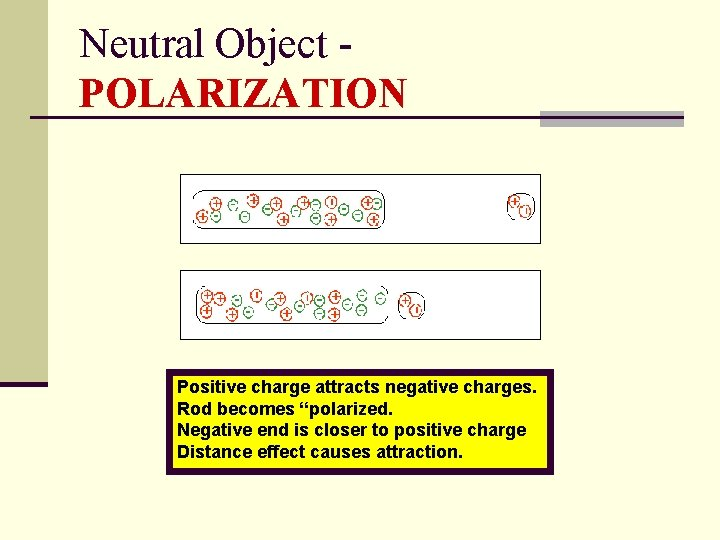Neutral Object POLARIZATION Positive charge attracts negative charges. Rod becomes “polarized. Negative end is closer to positive charge Distance effect causes attraction.InductionPolarize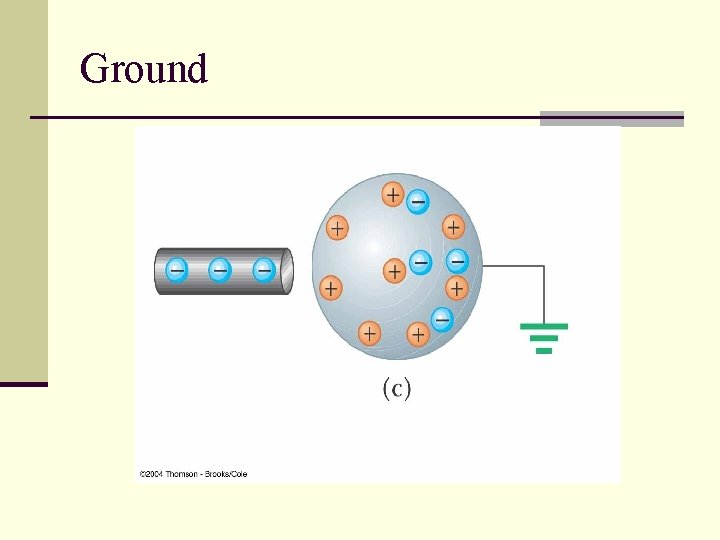Ground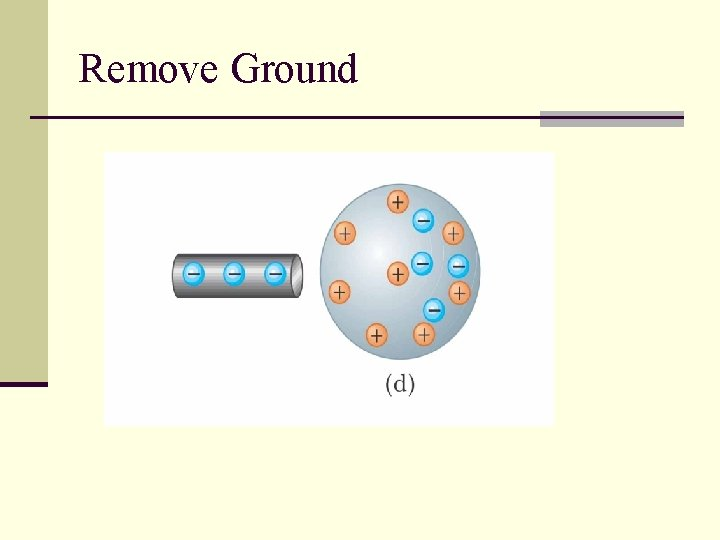Remove Ground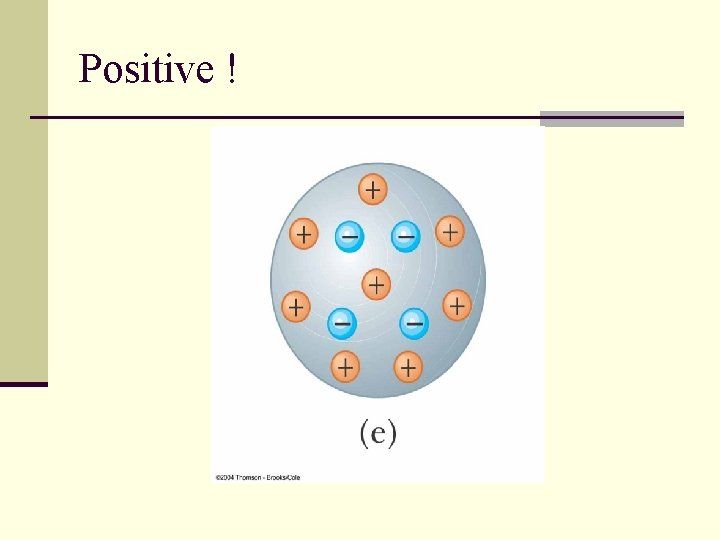Positive !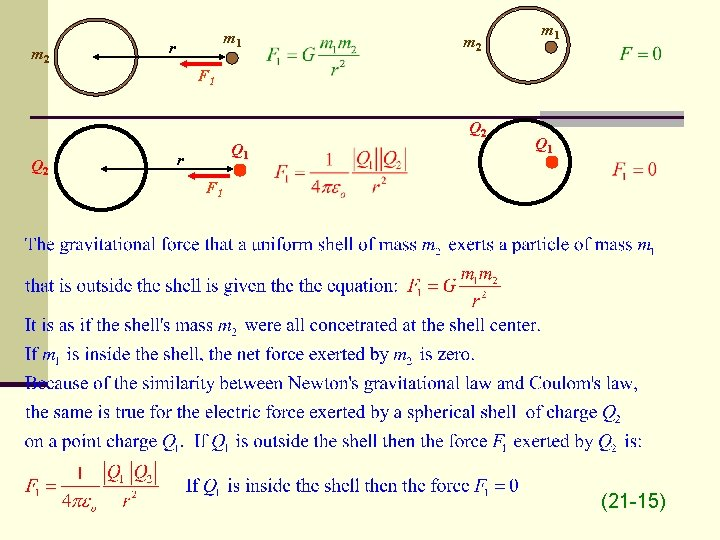m 2 m 1 r m 2 m 1 F 1 Q 2 Q 1 r Q 1 F 1 (21 -15)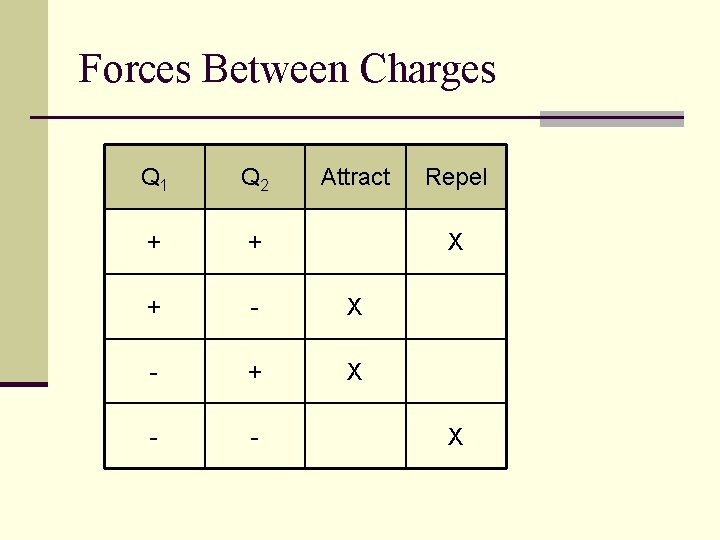Forces Between Charges Q 1 Q 2 Attract + + + - X - + X - - Repel X X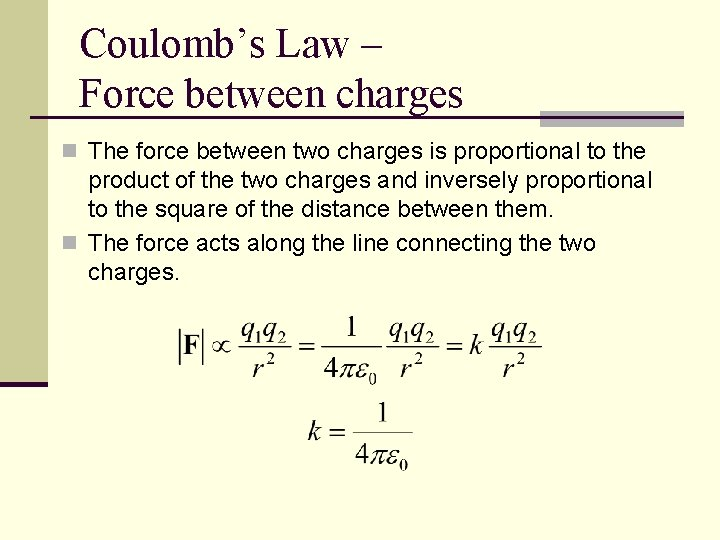Coulomb’s Law – Force between charges n The force between two charges is proportional to the product of the two charges and inversely proportional to the square of the distance between them. n The force acts along the line connecting the two charges.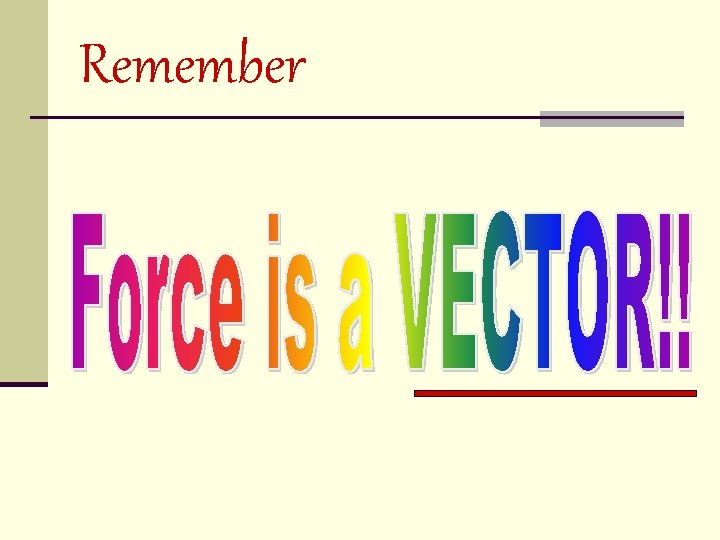Remember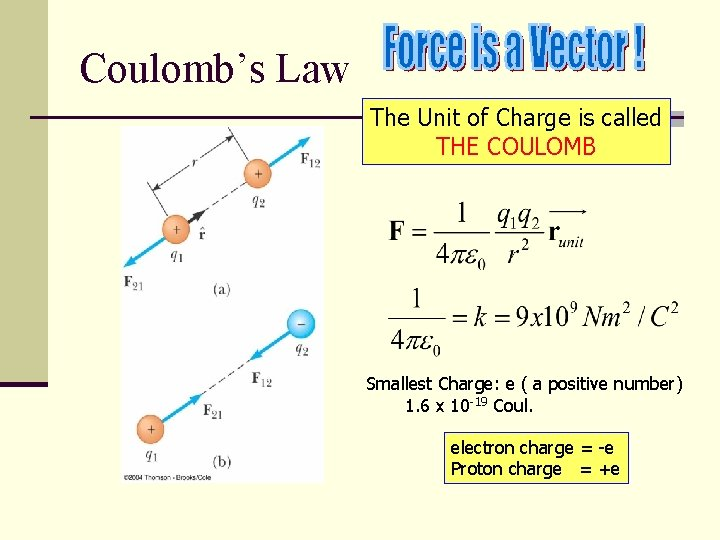Coulomb’s Law The Unit of Charge is called THE COULOMB Smallest Charge: e ( a positive number) 1. 6 x 10 -19 Coul. electron charge = -e Proton charge = +e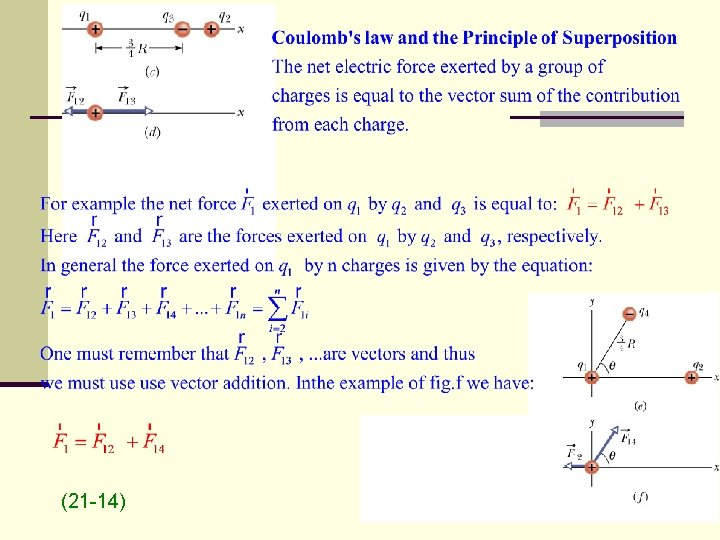(21 -14)m 2 m 1 r m 2 m 1 F 1 Q 2 Q 1 r Q 1 F 1 (21 -15)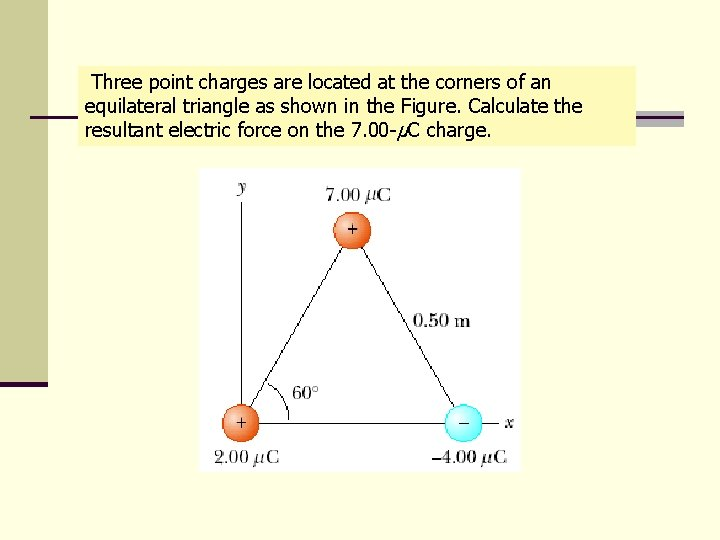Three point charges are located at the corners of an equilateral triangle as shown in the Figure. Calculate the resultant electric force on the 7. 00 -μC charge.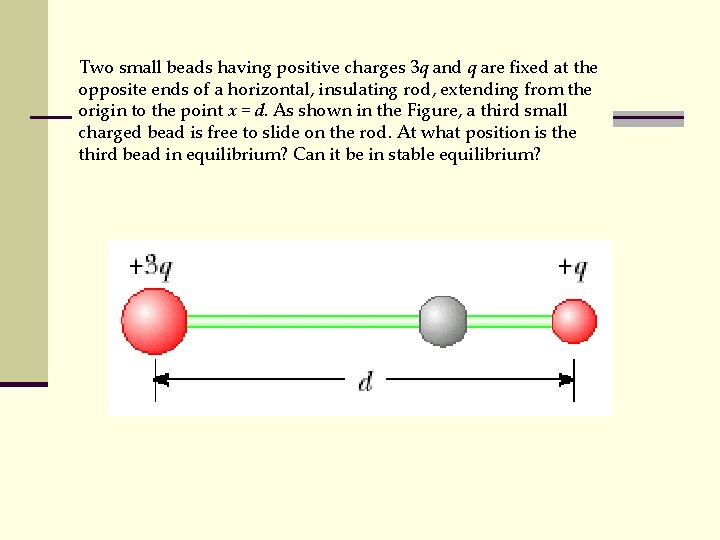Two small beads having positive charges 3 q and q are fixed at the opposite ends of a horizontal, insulating rod, extending from the origin to the point x = d. As shown in the Figure, a third small charged bead is free to slide on the rod. At what position is the third bead in equilibrium? Can it be in stable equilibrium?